# Theoretical Probability

Here we will learn about theoretical probability, including probability scales, mutually exclusive events and the probability of something not happening.

There are also theoretical probability worksheets based on Edexcel, AQA and OCR exam questions, along with further guidance on where to go next if you’re still stuck.

## What is theoretical probability?

Theoretical probability uses mathematics rather than an experiment to determine the chance of something happening.

To do this we need to think about two things – the number of times an event may occur and the total number of outcomes available.

\text{Theoretical probability }=\frac{\text{frequency of the event occurring}}{\text{total frequency of possible outcomes}}

Some events are known as mutually exclusive. This means they cannot happen at the same time.

For example, a fair dice cannot land on a 3 and a 4 at the same time and so the probability of this event would be 0 (impossible).

We may be asked to find the probability of rolling a 3 or a 4. As these events are mutually exclusive we can add the probabilities together. Here, it is stated that the dice is fair and so the probability of landing on each number is the same.

This means that P(3)=\frac{1}{6} and P(4)=\frac{1}{6} and P(3\text{ or }4)=\frac{1}{6}+\frac{1}{6}=\frac{2}{6}=\frac{1}{3}.

We can also place this value on a probability scale.

The probability scale goes from 0 (impossible) to 1 (certain). This means the probability of something not happening must be 1 subtract the probability of it happening.

We write P( not A) = 1-P(A).

The probability of the event occurring can be written as a fraction, decimal or percentage.

In probability theory, the difference between theoretical and experimental probability is that theoretical probability is based solely on pure maths, whereas experimental probability is based on the outcomes of an experiment and so there is no known probability until the experiment has taken place.

### What is theoretical probability?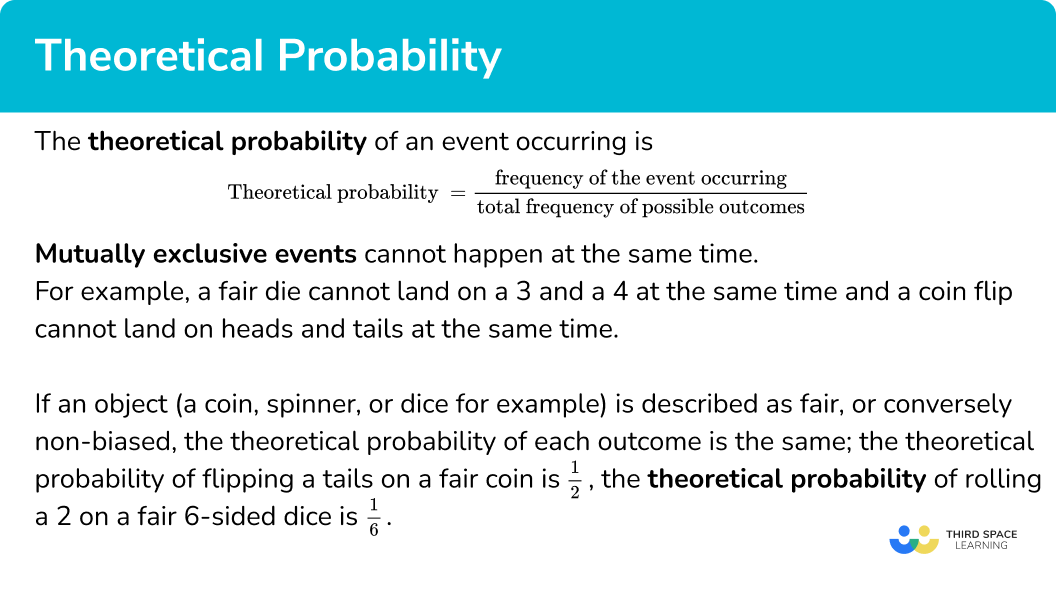## How to calculate the theoretical probability

In order to calculate the theoretical probability of an event:

1. Determine the frequency of the event occurring.
2. Determine the total frequency of all possible outcomes.
3. Substitute these values into the formula. Write your answer as a fraction, decimal or percentage.

\text{Theoretical probability = }\frac{\text{frequency of the event occurring}}{\text{total frequency of possible outcomes}}

### Explain how to calculate the theoretical probability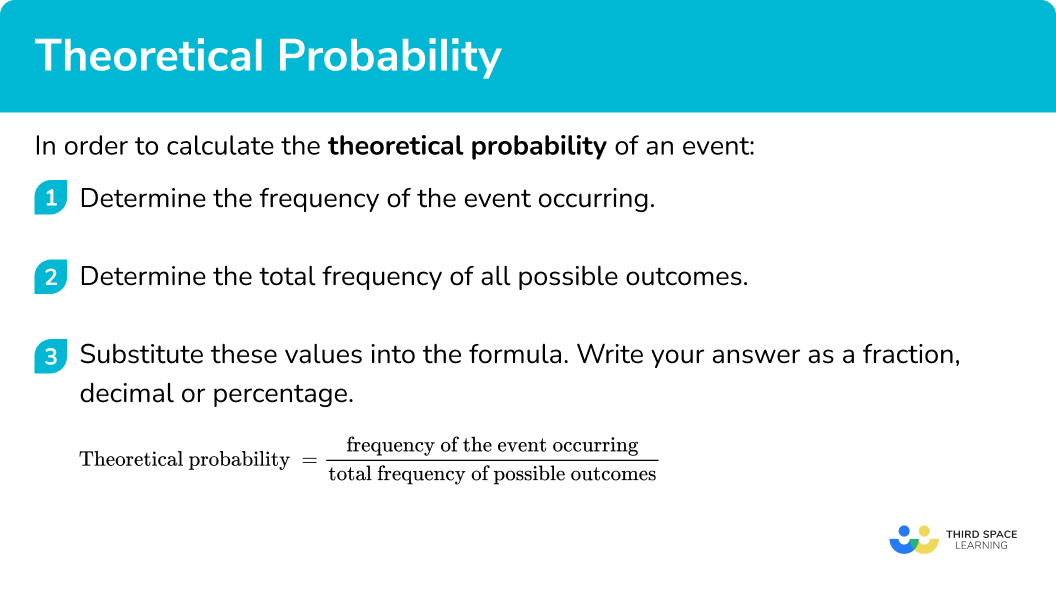### Related lessons onprobability distribution

Theoretical probability is part of our series of lessons to support revision on probability distribution. You may find it helpful to start with the main probability distribution lesson for a summary of what to expect, or use the step by step guides below for further detail on individual topics. Other lessons in this series include:

## Theoretical probability examples

### Example 1: selecting a random letter

Lettered cards spell out the word mathematics.

The cards are mixed up and one is selected at random.

What is the probability that the card selected is a vowel?

1. Determine the frequency of the event occurring.

The set of vowels are: A, E, I, O, and U. There are \bf{4} vowel cards in the word MATHEMATICS.

2Determine the total frequency of all possible outcomes.

There are 11 cards altogether in the word MATHEMATICS.

3Substitute these values into the formula. Write your answer as a fraction, decimal or percentage.

As we are calculating the theoretical probability of a vowel, where the frequency of the event occurring is 4 and the total frequency of possible outcomes is 11, we can write

P(\text{Vowel})=\frac{4}{11}.

### Example 2: rolling a number on a fair die

A fair six-sided die is rolled. What is the probability of it landing on a prime number?

A list of prime numbers gives, 2, 3, 5, 7, 11, …

The prime numbers on a fair six-sided die are 2, 3, and 5.

Therefore the frequency of prime numbers is 3.

As the die is six-sided, the total number of outcomes from a single roll is 6.

P(\text{Prime number})=\frac{3}{6}=\frac{1}{2} \ (=0.5)

### Example 3: selecting a counter from a bag

A bag contains 15 red counters and 5 green counters. A counter is selected from the bag at random. Find the probability of the counter being red and display it on the probability scale.

The frequency of red counters is 15.

The total number of counters in the bag is 20.

P(\text{Red})=\frac{15}{20}=\frac{3}{4} \ (=0.75)

Placing this on a probability scale, we have

### Example 4: event not occurring

A bag contains 6 yellow counters, 3 blue counters and 1 white counter. A counter is selected from the bag at random. Find the probability that the counter is not yellow.

The frequency of yellow counters is 6.

The total number of counters in the bag is 10.

P(\text{Yellow })=\frac{6}{10}=0.6

As the events are mutually exclusive, the probabilities will all add to 1.

The probability of not picking a yellow counter can therefore be found by subtracting this answer from 1. As we can use the fraction or decimal equivalent, here are both methods.

Using decimals,

P(\text{Not Yellow })=1-0.6=0.4

Using fractions,

P(\text{Not Yellow })=1-\frac{6}{10}=\frac{4}{10}.

### Example 5: union of two mutually exclusive events

A bag contains 6 yellow counters, 3 blue counters and 1 white counter. A counter is selected from the bag at random. Find the probability of the counter being yellow or white.

The frequency of yellow counters is 6 and the frequency of white counters is 1. This means that the frequency of yellow or white counters is 6+1=7.

The total number of counters in the bag is 10.

P(\text{Yellow or White })=\frac{7}{10}=0.7

### Example 6: intersection of two events

A coin is flipped twice. What is the probability that the coin lands on tails both times?

A coin can land on either heads or tails. The frequency of a tails is therefore 1.

There are two possible outcomes (heads or tails) and so the total frequency is 2.

P(Tails)= \frac{1}{2}.

As we want to determine the probability of a tails twice, we need to multiply the probability of a tails in flip 1, by the probability of a tails in flip 2. This gives us

P(\text{Tails and Tails })=\frac{1}{2}\times\frac{1}{2}=\frac{1\times{1}}{2\times{2}}=\frac{1}{4}.

### Common misconceptions

• The probability of rolling a number on a fair die is equal to that number over \bf{6}

A common error when asking for the probability of getting a 2 on a fair die is \frac{2}{6}.

It is important to remember that if the die is fair, all outcomes will be equally likely so in this case the probability of getting any of the numbers 1 to 6 on a fair die is \frac{1}{6}.

• Probabilities can be expressed using a ratio

It is very common to hear probabilities described using ratios, “there is a 3:1 chance of something happening”. For the purposes of GCSE mathematics, probabilities should only be expressed as fractions, decimals or percentages.

• Frequency over remaining frequency

Similarly to writing the answer to a probability question as a ratio, the fraction is written as the frequency of the event occurring out of the remaining frequency. For example, the probability of rolling a 4 on a fair six-sided dice is 1 out of 5 as there are 5 numbers remaining.

This is incorrect. The correct answer is 1 out of 6 because the probability is equal to the frequency of the event occurring, 1, divided by the total number of possible outcomes, 6.

### Practice theoretical probability questions

1. Lettered cards spell out the word fraction.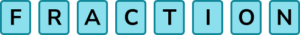The cards are mixed up and one is selected at random. Determine the probability that the card selected is a vowel.

\frac{5}{8}\frac{3}{8}\frac{1}{3}\frac{1}{2}There are 3 vowels and 8 lettered cards in total and so

P(\text{Vowel})=\frac{3}{8}.

2. Cards numbered 1 to 10 are mixed up and one is selected at random.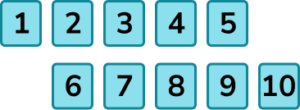What is the theoretical probability that the card selected is a square number?

\frac{3}{7}\frac{2}{10}\frac{3}{10}\frac{1}{3}There are 3 square numbers (1, 4, 9) and 10 numbered cards in total.

3. A bag contains 5 red counters and 4 blue counters. A counter is selected at random. What is the probability that the counter is blue?

\frac{5}{9}\frac{4}{5}\frac{1}{2}\frac{4}{9}There are 4 blue counters and 9 counters in total.

4. A bag contains 3 green counters, 5 white counters and 4 red counters. A counter is selected at random. Calculate the probability of the counter being green or red.

\frac{7}{12}\frac{5}{12}\frac{1}{12}\frac{2}{3}There are 7 green and red counters and 12 counters in total.

5. A bag contains 3 green counters, 5 white counters and 4 red counters. A counter is selected at random. Calculate the probability of the counter that is selected is not green. Display your answer on a probability scale.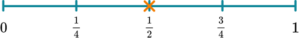There are 3 green counters and 12 counters in total.

1-\frac{3}{12}=1-\frac{1}{4}=\frac{3}{4}

6. A six-sided spinner shows only the numbers 1, 2, 3 and 4.

There is an equal chance of it landing on a 2 or a 4, but more of a chance of it landing on an odd number than an even number.

Select the spinner that satisfies those probability statements.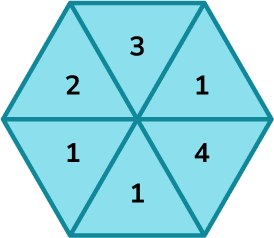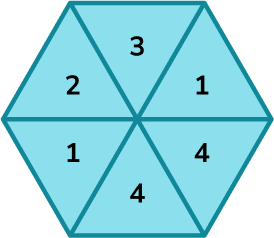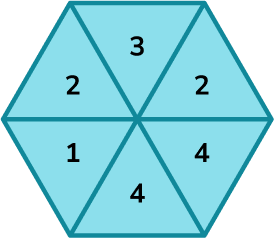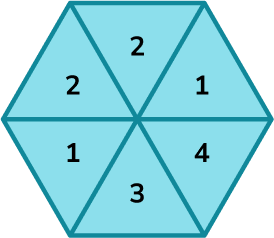There must be the same number of 2s and 4s. There must be more odd numbers than even numbers and so the remaining values must all contain either a 1 or a 3 but not the same number of 1s and 3s.

### Theoretical probability GCSE questions

1. A weather report states that there is a 30\% probability of rain. What is the probability of it not raining?

(2 marks)

100\%-30\% or 1-0.30

(1)

70\% or 0.7 or equivalent

(1)

2. On each probability scale, mark with a cross (X) the probability for each statement.

(a) The probability of flipping a fair coin and it will show tails.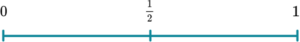(b) The probability of selecting a red ball from a bag containing 2 green balls, 5 red balls, 7 blue balls and 6 white balls.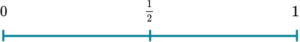(c) The probability of rolling a multiple of 10 on a standard six-sided die.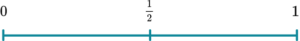(3 marks)

(a)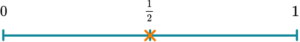(1)

(b)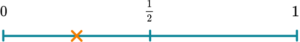(1)

(c)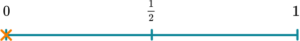(1)

3. A bag contains 30 counters. 7 are red, 11 are green and the rest are blue or white.

There are twice as many blue counters as white counters.

A counter is selected at random.

Find the probability of selecting a blue counter.

(2 marks)

(12 \div 3) \times 2=8 blue

(1)

\frac{4}{30} or equivalent

(1)

## Learning checklist

You have now learned how to:

• Record, describe and analyse the frequency of outcomes of simple probability experiments involving randomness, fairness, equally and unequally likely outcomes, using appropriate language and the 0-1 probability scale
• Apply the property that the probabilities of an exhaustive set of mutually exclusive events sum to one

## Still stuck?

Prepare your KS4 students for maths GCSEs success with Third Space Learning. Weekly online one to one GCSE maths revision lessons delivered by expert maths tutors.

Find out more about our GCSE maths tuition programme.